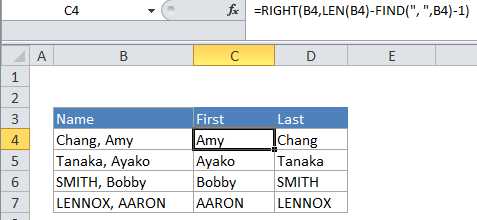# Get first name from name with comma — Manipulating NAMES in Excel

To extract the first name from a full name in “Last, First” format, you can use a formula that uses RIGHT, LEN and FIND functions.

Note: this formula will only work with names in Last, First format, separated with a comma and space.

## Formula

`=RIGHT(name,LEN(name)-FIND(", ",name)-1)`## Explanation

From the table and formula (above), name represent full name in this format:

LAST, FIRST
Philip, White
Tom, Green
Chalk, Black

A comma and space separate the last name from the first name.

Worked Example:   How to translate letters to numbers in Excel

In the example, the active cell contains this formula:

`=RIGHT(B4,LEN(B4)-FIND(", ",B4)-1)`

### How this formula works

At a high level, this formula uses RIGHT to extract characters from the right side of the name. To figure out the number of characters that need to be extracted to get the first name, the formula uses the FIND function to locate the position of “, ” in the name:

`FIND(", ",B4) // position of comma`

FIND returns the position of the comma and space as a number. This number is then subtracted from the total length of the name:

`LEN(B4)-FIND(", ",B4) // length of first name + 1`

The result is the length of the first name, plus one extra character, due to the comma. To get the true length, 1 is subtracted:

`LEN(B4)-FIND(", ",B4)-1 // length of the first name`

Because the name is in reverse order (LAST, FIRST), the RIGHT function can simply extract the length of the first name.

Worked Example:   How to Separate Text Strings in Excel

For the example, the name is “Chang, Amy”, the position of the comma is 6. So the inner formula simplifies to this:

Worked Example:   How to split text string at specific character in Excel

10 – 6 – 1 = 3 // length of first name

Then:

`RIGHT("Chang, Amy",3) // "Amy"`